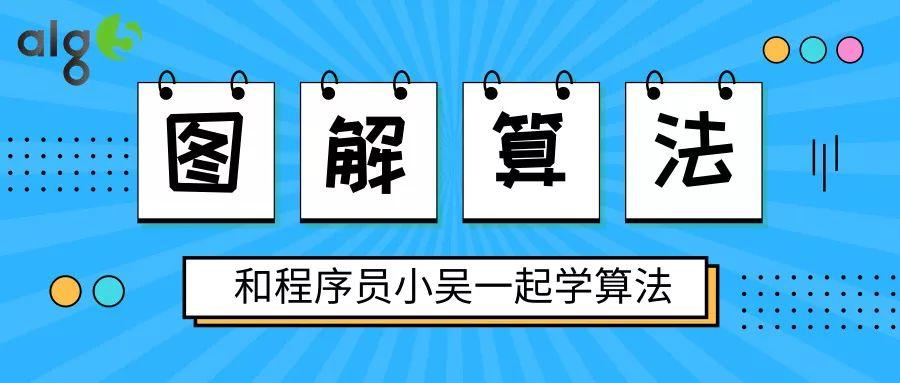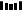### 题目描述

输入：A = [34,23,1,24,75,33,54,8], K = 60输出：58解释：34 和 24 相加得到 58，58 小于 60，满足题意。

输入：A = [10,20,30], K = 15输出：-1解释：我们无法找到和小于 15 的两个元素。

1. 1 <= A.length <= 100

2. 1 <= A[i] <= 1000

3. 1 <= K <= 2000

### 代码实现

public int twoSumLessThanK(int[] A, int K) {    if (A == null || A.length == 0) {        return -1;    }    Arrays.sort(A);    int l = 0, r = A.length - 1;    int result = Integer.MIN_VALUE;    while (l < r) {        if (A[l] + A[r] >= K) {            r--;        } else {            result = Math.max(result, A[l] + A[r]);            l++;        }    }    return result == Integer.MIN_VALUE ? -1 : result;}1.程序员

2.【GitHub

3.【算法动画：七分钟理解什么是KMP算法

4.【数据结构十大经典排序算法动画与解析，看我就够了！